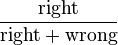# Grade (education) facts for kids

Kids Encyclopedia Facts

Grades are a teacher's evaluation of a student's performance. They are often letters (e.g. A, B, C, D, F,) or numbers (e.g. 1-10.), although in some countries they do not use either of these systems.

Letter grades are most commonly used in the United States and some others. The grades D or C are usually enough to pass.

Grades can also be on a number scale. Typically a midpoint score is a pass. Sometimes the numbers can go in descending order to indicate better performance.

A Grade Point Average, or GPA, takes the grade(s) of a student and assigns point values to them. These points are added up and divided to calculate the GPA.

Simple grades can be found with this formula:$\frac{\text{right}}{\text{right}+\text{wrong}}$

The grade is the answer to that formula turned into a percent.

This is the type of grading the United States uses the most:

90%-100% A+
93%-96% A
90%-92% A-
87%-89% B+
83%-86% B
80%-82% B-
77%-79% C+
73%-76% C
70%-72% C-
67%-69% D+
63%-66% D
60%-62% D-
0%-59% FGrade (education) Facts for Kids. Kiddle Encyclopedia.